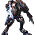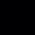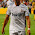### 6 AM Quiz (Problem Solving) Is the problem obvious?

Do you think Mr Kanbili is fair and Mr Ong should accept the suggestion?
1. What is your stand?
2. Give an example to illustrate your stand.
3. Explain how you come to this conclusion (Hint: Use algebra).

Note that the quiz is not a compulsory activity. It serves to stretch our mind a little to see how we apply what we learn in classrooms in real world :)

#### 38 comments:

1.1.Mr Kanbili is not being fair

2.If the north is 30 m and east 45m , the area is NOT 1600m

3. a x a ≠ a x b
a^2 ≠ ab

1.Good attempt to use algebra,
However needs to relate you explanation to the context.
Try to elaborate.

2.1) I think Mr Kanbili is not fair and that Mr Ong should not accept the suggestion.

2) If the one going north gets a little bit shorter and becomes 1m, while the one going east becomes 79m, then the area of the land that Mr Ong gets is 79 square metres and not 1600 square metres.

3) This is scenario one where you get a square piece of land. Both sides are of the same length, so, a x a = 2a
This is scenario two where you get the rectangular piece of land. The both sides are not the same in length but the total is the same,
so, a-1 x a+1 = 2a-1
Now you can see in the algebraic forms that the total area of land does not remain the same.

1.ur algebra fail :p a x a is not 2a it s a^2
:D

2.Good attempt.

As Gabriel pointed out, there's error when you simplify the algebraic expression.
Try again.

3.Mr kanbili is not being fair.
lets take the length of one side of the square as a(40m).
a^2 will be the area that was agreed on(1600m^2).
lets take b as the length that was subtracted from the side going north and the length added to the side going east.
So, the area of the rectangle field is (a-b)(a+b) which is a^2-b^2
since a^2-b^2 is smaller than a^2,Mr kanbili is not fair.
:)

1.Good explanation.
You've used algebra to generalize the explanation.
How could this be further improved?

4.1)I think that Mr Kanbili is not fair.
2)If one North becomes 35m and the East becomes 45m, then the area will be 1350m^2 not 1600m^2.
3)a x a=a^2(Scenario 1 whereby the land is squarish.)
a-5 x a+5= a^2-25(Scenario 2 whereby the land is in a rectangle shape.

1.Good attempt to use algebra.
Now, how can we generalize the explanation without testing the sides with different lengths?

5.I think it is correct :D

6.1) Mr Kanbili is not fair and Mr Ong should reject the proposal.

2) If the length is decreased by 5m and the breadth is increased by 5m, then

(40-5)(40+5) = 1575 square metres.
We can clearly see now that an increase in breadth and a decrease in length is not equal to 1600 square metres.

3) Let us take 'x'= 40m and 'y'= 40m

x*y = 1600 square metres

However, in Mr Kanbili's explanation,
(Let 'a' be the increase and decrease in the breadth and length respectively)

Length= (x-a)
Breadth= (y+a)

Thus,

(x-a)(y+a)= [xy+ax-ay-(a^2)] square metres
[xy+ax-ay-(a^2)] square metres ≠ (xy) square metres

Hence, Mr Kanbili is not fair

1.Sorry for the first line of Q3 it should be: 'x' represents the length and 'y' represents the breadth

2.Based on your 'generalisation', we can only tell that the new area may vary from the original, however, it was non-conclusive.

3.Suggestion: relook at the given condition "square"

7.1)I think that Mr Kanbili is not fair.
2) If the length is decreased by 5m and the breadth is increased by 5m, then (40-5) x (40+5) = 1575 square metres.
therefore, we can say that there is a difference between the two areas.
3) For example,take a as 40 and b as 35.
a x a is not equal to a x b

1.From the example, make an attempt to generalize.

8.1) I think Mr Kanbili is not being fair.
2) If the length is decreased by 10m going north and it is increased by 10m going east then it would be,
(30m-10m)(30m+10m) = 20m x 40m = 800m^2 ≠ 1600m^2
3) Lets take 40m as 'a' and the distance short of and excess of on the north and east respectively as 'b'
Length of the field = (a-b)
Breadth of the field = (a+b)
So the area would be,
(a-b)(a+b) = a^2 + ab - ab - b^2
= a^2 - b

The actual area should be a x a = a^2
This means the field is 'b' meter square lesser than the real amount.

1.Good explanation till... Towards the end, why "b^2" became "b"?

9.I think that Mr Kanabili is not fair

supposed to be
40x40=1600

north length= x
east length= x

later
a = added length

(x-a) x (x+a) = x^2 + xa + -ax -a^2, which is x^2 -a^2

if x is 40, and a is 20,

so x^2 -a^2 will be 1600 - 400, which is 1200.

As long as a is >40, the amount of land is lesser than what it was supposed to be.

1.The example has presented the situation correctly.
However, why did you conclude that as long as a > 40, the land is lesser (as a is the added length)?
Why this magic number?

10.1. What is your stand?
I think Mr Kanbili is not fair.

2. Give an example to illustrate your stand.
Given that the original dimensions of the land was 40m by 40m,
(40-5)m * (40+5)m = 1575m^2 ≠ 1600m^2

3. Explain how you come to this conclusion (Hint: Use algebra).
Take length to be a, breadth to be b.
Original area : a*b=ab
New area(aft. change) : (a-5)(b+5)=ab+5a-5b-25
≠ab
Therefore Mr Kanbili is not being fair.

1.For Part (3), you could possibly consider to use a variable (e.g. "x") to represent the 'change' in length to help explain.

11.1. I think Mr Kanbill is not fair.

2. (40-10)m x (40+10)m ≠ 1600m^2

3. (a-B) x (A+B) = A^2-B^2, A x A = A^2

1.What can you conclude from Parts (2) & (3)?

12.This comment has been removed by the author.

13.1. What is your stand?
I feel that Mr Kanbill is not being fair.
2. Give an example to illustrate your stand.
36x40=1440
40x40=1600
The area of the perfect square is larger than that of the rectangle.
3. Explain how you come to this conclusion
Assuming that a is the vertical length while B is the horizontal length, if a is 4m(a is 2m less than 40m while b is 2m more than 40m) shorter than b, (b+2)(a-2) = ab+2a-2a-2b. However, if a b are of equal length, it would be a(b) = ab, with nothing subtracting ab, thus the area of the perfect square would of course be larger.

1.For Part (3), it sounds a bit confusing.
Let's look at (b+2)(a-2) = ab+2a-2a-2b again. Check if there's any error in the expansion?

14.1) Mr Kanbili is not fair

2)Mr Kanbili is wrong as when north side is less and the east side is more at an equal distance it does not have a same area as (40-5)(40+5) = 1575 square metres while 40^2 1600m

3)If a is 40 and b is the more and less portion (a-b)(a+b) = a^2 + ab - ab - b^2= a^2 - b and when a and a is multiplied it is a^2 it is more than a^2 - b

1.Will have to address the error before explaining further:
(a-b)(a+b) = a^2 + ab - ab - b^2= a^2 - b

15.1) I think that mr Kanbill is not fair.

2) Before:
North Length=x
South length=x
Area=x^2

After (one with length added and one with length decreased):
North Length=x+y
South Length=x-y
Area=(x+y)(x-y)
=x^2 - y^2

3) As shown above, the areas are different. Thus, Mr Kanbill cannot say that the areas are the same and therefore he is not fair.

1.Good and clear explanation :)

2.I think the example is missing?

16.1) I think that Mr Kanbill is not being fair.

2) If both sides are the same length : Area -> a * a = a^2
If both sides are not of the same length : Area -> (a - b) * (a + b) = a^2 + ab - ab - b^2 = a^2 - b^2. Therefore the area will be lesser.

Examples:
Let a be 40.
Area : a^2 = 40 * 40 = 1600m^2
If it is short on one side and longer on the other,
Let x be 35 and y be 45.
Area : x * y = 35 * 45 = 1575m

3) If both sides are not equal, the area will be lesser than when both sides are the same so Mr Kanbill is not being fair.

1.Good and clear explanation :)

17.1) I think that Mr Kanbill is not being fair.

2) Original:
North length- a
East length- a
Area: a^2

Now:
North length- a-n
East length- a+n
Area: (a-n)(a+n)= a^2-n^2

a^2-n^2 ≠ a^2

3) As shown from the above, the areas are not the same. The area now is lesser then the original area.

1.Good and clear explanation.
Use numbers to give an illustration (example).

18.1. What is your stand?
I think MR Kanbill is not being fair.

2. Give an example to illustrate your stand.
For example, if the area is actually a 9 by 9, the original area will be 81m^2. But if we take away 2 from one side and add 2 to the other, we get 7x11=77m^2 hence the two areas are different.

3. Explain how you come to this conclusion

X x X=X^2
(X-2)x(X+2)=X^2+2X-2X-4
=X^2-4

19.You could make reference to the original context given (square piece of land with size 40m).
For generalisation, you can let a variable be the 'change' (to explain the difference)